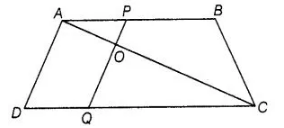# In figure, if AB || DC and AC,

Question:

In figure, if AB || DC and AC, PQ intersect each other at the point 0. Prove that OA . CQ = 0C . AP.Solution:

Given AC and PQ intersect each other at the point O and AB || DC

Prove that OA . CQ = 0C . AP

Proof $\ln \Delta A O P$ and $\Delta C O Q$, $\angle A O P=\angle C O Q \quad$ [vertically opposite angles]

$\angle A P O=\angle C Q O$

[since, $A B \| D C$ and $P Q$ is transversal, so alternate angles]

$\therefore \quad \Delta A O P \sim \Delta C O Q \quad$ [by AAA similarity criterion]

Then, $\frac{O A}{O C}=\frac{A P}{C Q}$ [since, corresponding sides are proportional]

$\Rightarrow$ $O A \cdot C Q=O C \cdot A P$ Hence proved.